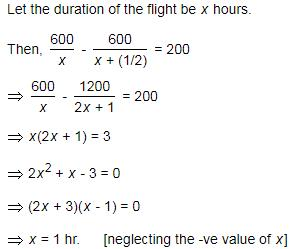Courses

# Test: Speed, Time & Distance- 2

## 10 Questions MCQ Test IBPS Clerk Prelims - Study Material, Mock Tests | Test: Speed, Time & Distance- 2

Description
This mock test of Test: Speed, Time & Distance- 2 for Quant helps you for every Quant entrance exam. This contains 10 Multiple Choice Questions for Quant Test: Speed, Time & Distance- 2 (mcq) to study with solutions a complete question bank. The solved questions answers in this Test: Speed, Time & Distance- 2 quiz give you a good mix of easy questions and tough questions. Quant students definitely take this Test: Speed, Time & Distance- 2 exercise for a better result in the exam. You can find other Test: Speed, Time & Distance- 2 extra questions, long questions & short questions for Quant on EduRev as well by searching above.
QUESTION: 1

### A man goes to his office from his house at a speed of 3 km/hr and returns at a speed of 2 km/hr. If he takes 5 hours in going and coming, what is the distance between his house and office?

Solution:

If the distance between house and office is d km
Then time taken to go from home to office = d/3 hr (Time = Distance/Speed)
and Time taken to come back from office to home= d/2 hr
So total time taken = (d/3 +d/2) hour
But according to question , time taken = 5 hour
so we have
d/3+d/2=5
or (2d+3d)/6= 5
or 5d/6=5
or, d=5 x6/5= 6km
So distance between home and office = 6km

QUESTION: 2

Solution:
QUESTION: 3

### A Man travelled a distance of 61 km in 9 hours. He travelled partly on foot at 4 km/hr and partly on bicycle at 9 km/hr. What is the distance travelled on foot?

Solution:

Let the time in which he travelled on foot = x hour
Time for travelling on bicycle = (9 - x) hr

Distance = Speed * Time, and Total distance = 61 km
So,
4x + 9(9-x) = 61
=> 5x = 20
=> x = 4

So distance traveled on foot = 4(4) = 16 km

QUESTION: 4

It takes eight hours for a 600 km journey, if 120 km is done by train and the rest by car. It takes 20 minutes more, if 200 km is done by train and the rest by car. What is the ratio of the speed of the train to that of the car?

Solution:

Let the speed of the train be x km/hr and that of the car be y km/hr.
It takes eight hours for a 600 km journey, if 120 km is done by train and the rest by car.
120/x + 480/y = 8 or 1/x + 4/y = 1/15
If 200 km is done by train and the rest by car, then 20 minutes more time is taken.
200/x + 400/y = 25/3 or 1/x + 2/y  = 1/24
Solving both the equations,
x = 60 and y = 80
Ratio is 3 : 4

QUESTION: 5

In a flight of 600 km, an aircraft was slowed down due to bad weather. Its average speed for the trip was reduced by 200 km/hr and the time of flight increased by 30 minutes. What is the duration of the flight ?

Solution:option "D"

QUESTION: 6

If a person walks at 14 km/hr instead of 10 km/hr, he would have walked 20 km more. What is the actual distance travelled by him?

Solution:

Distance he could travelled/speed diff.
= 20/(14-10)
= 20/4
= 5 hrs
Now his actual speed was 10 km/h
Total distance travelled by him = speed × time
= 10 × 5
= 50 km.

QUESTION: 7

A man rides his bicycle 10 km at an average speed of 12 km/hr and again travels 12 km at an average speed of 10 km/hr. What is his average speed for the entire trip approximately?

Solution:

Total Distance = 10 +12 = 22 km
Total time = 10/12 + 12/10 = 5/6 + 6/5 = 25/30 + 36/30 = 61/30 hours
Average speed = 22 /(61/30) = 660/61 = 10.8 km/ hour

QUESTION: 8

The ratio between the speeds of two trains is 7 : 8. If the second train runs 400 km in 4 hours, What is the the speed of the first train?

Solution:
QUESTION: 9

Walking 6/7th of his usual speed, a man is 12 minutes too late. What is the usual time taken by him to cover that distance?

Solution:

Let the distance be x
Let the original speed be y
Time originally taken = distance / speed
That means = x/y
Now the new speed is (6/7)y
And new time is distance /new speed
x/(6/7)y that can be written as 7x/6y
Now new time - original time = 12 min
7x/6y-x/y=12
After subtracting we get
x/y = 12×6
x/y = 72min

QUESTION: 10

It takes eight hours for a 600 km journey, if 120 km is done by train and the rest by car. It takes 20 minutes more, if 200 km is done by train and the rest by car. What is the ratio of the speed of the train to that of the car?

Solution: